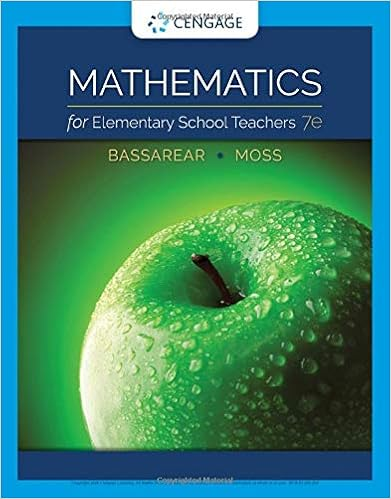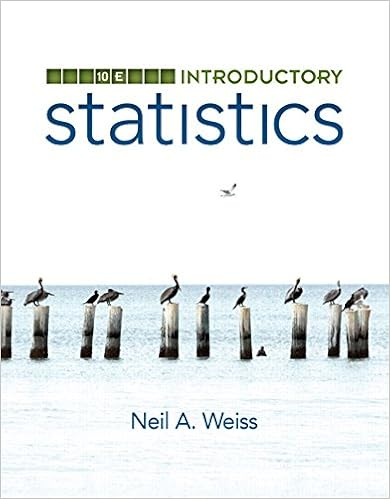# Linear systems in two unknowns we will also sometimes

• Lab Report
• 19
• 54% (26) 14 out of 26 people found this document helpful

This preview shows page 10 - 13 out of 19 pages.

LINEAR SYSTEMS IN TWO UNKNOWNSWe will also sometimes need to solvepairs oflinear equations intwounknowns. Forexample, suppose that two unknown numbersxandysatisfyand.How can we findxandy,other than by tedious trial and error?Here's one way: Solve the first equation foryin terms ofx,using the method discussed in thepreceding section: we get.Then plug this into the second equation:Solve this forx:we getx=5.Now plug back into the equation:we get.So we have the answer:x=5, y=8. Now check this answer by plugging intothe two original equations and making sure that it satisfiesbothof them.(Do it!!)Don't skip this last step: it's a crucial way of catching one's arithmetic or algebra mistakes,and it's well worth the extra time it takes.This technique always works: Pickeither one ofthe equations and solve it foreither oneofthe variables in terms of the other; then plug into the remaining equation, solve it, and plugback. Which equation and which variable to choose? It doesn't matter; pick whichever oneseems to make the arithmetic simplest.You may also remember from high-school algebra some clever tricks for solving systems oflinear equations (e.g. adding or subtracting the equations). Feel free to use these tricks if youfind that it speeds up your computation; you can always fall back on the method illustratedabove, if nothing else works. Whatever method you use, don't forget to check your answer atthe end!
##### We have textbook solutions for you!The document you are viewing contains questions related to this textbook.
Chapter 5 / Exercise 33
Mathematics for Elementary School Teachers
BassarearExpert VerifiedBrowse all Textbook Solutions
Math Review11TRIGONOMETRYIn this course we will also need, from time to time, some elementary trigonometry.4Consider the small right triangle in the picture below, whose sides have lengthsa,bandc.Consider also the larger right triangle, whose sides have lengthsA, Band C. These two righttriangles aresimilar triangles,so theratios ofthe sides are the same in both:b/a = B/A, c/a=C/Aand so forth. Therefore, these ratios depend only on the angle, not on the sizeofthetriangle. We call themtrigonometric functions ofthe angle, and give them the namessine,cosineandtangent:4From Greektrigonon, triangle +metric,measurement.
Math Review12A useful mnemonic is SOH—CAH—TOA: sine = opposite/hypotenuse, cosine =adjacent/hypotenuse, tangent = opposite/adjacent. Note that since the sine, cosine andtangentofthe angleφare ratiosoflengths, they aredimensionless,i.e. pure numbers thatdon't depend on the units being used. For example, if,and, wehave:and we'd get the same answer sin0= 0.6 if we first converted the lengthsa, band c to inchesor kilometers or furlongs.You're probably familiar with measuring angles in the ancient Babylonian system ofdegrees,in which the whole circle equals 360 degrees (360°). Another system, used in nearly alladvanced work, measures angles inradians:arcs of a circle are measured in units of thecircle's radius, so that the whole circle equals 2πradians. To convert between degrees andradians, observe that 360 degrees = 2πradians, so that 1 radian = 360/(2π) degrees57.296degrees.

Upload your study docs or become a

Course Hero member to access this document

Upload your study docs or become a

Course Hero member to access this document

End of preview. Want to read all 19 pages?

Upload your study docs or become a

Course Hero member to access this document

Term
Fall
Professor
Jordan
Tags
Logarithm
##### We have textbook solutions for you!
The document you are viewing contains questions related to this textbook.The document you are viewing contains questions related to this textbook.
Chapter 5 / Exercise 33
Mathematics for Elementary School Teachers
BassarearExpert Verified
•••